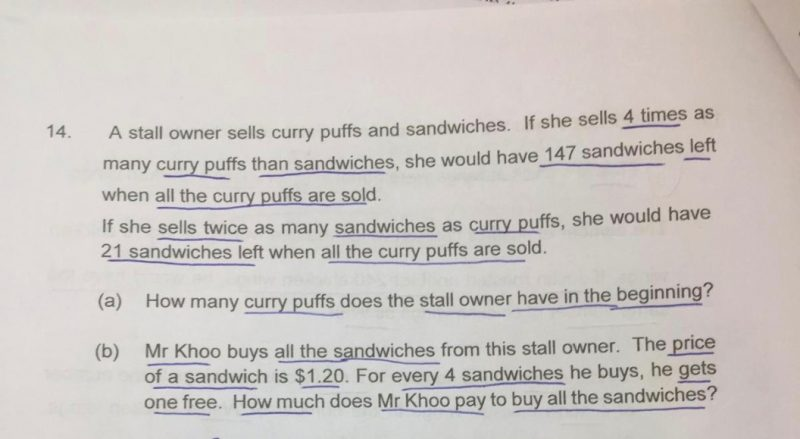# QuestionCurry Puff Sold (= total curry puff) Sandwich Sold Sandwich left Total Sandwich Scenario 1 4u 1u 147 1u + 147 Scenario 2 1p 2p 21 2p + 21

From Curry Puff, we can see that

4u = 1p

From Sandiwch, we can see that

1u + 147 = 2p + 21

1u + 147 = 2(4u) + 21

1u + 147 = 8u + 21

7u = 126

1u = 18

(a) Total curry puffs -> 4u = 4 × 18 = 72

Total sandwiches -> 8u + 21 = 144 + 21 = 165

Mr Khoo paid for 4 sandwiches to get 5 sandwiches.

Every 5 sandwiches cost 4 × \$1.20 = \$4.80

165 ÷ 5 = 33

In total, Mr Khoo paid 33 × \$4.80 = \$158.40